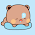Friday, July 10, 2009

Binary GCD algorithm

Here is a sample code for calculating GCD bitwise:
`typedef unsigned int ui;ui gcd(ui u, ui v){    ui shift, diff;        if (u == 0 || v == 0)        return u | v;        /* Let shift := lg K,    where K is the greatest power of 2 dividing both u and v. */    for (shift = 0; ((u | v) & 1) == 0; ++shift)    {        u >>= 1;        v >>= 1;    }        while ((u & 1) == 0)        u >>= 1;            /* From here on, u is always odd. */    do {        while ((v & 1) == 0)            v >>= 1;                    /* Now u and v are both odd, so diff(u, v) is even.        Let u = min(u, v), v = diff(u, v)/2. */        if (u < v)            v -= u;        else        {            diff = u - v;            u = v;            v = diff;        }        v >>= 1;        } while (v != 0);        return u << shift;}`

Actually, this algorithm outperforms the basic euclidean algorithm, so their asymptotic performance are same. According to Wikipedia, it is sometimes 60% faster than normal euclidean algorithm [proven].

But who needs it when he can do the following:
`int gcd(int a, int b){    while(b) b ^= a ^= b ^= a %= b;    return a;}`

Stunned? oh that's normal...

1.btw, who will ever need this... only good for assembly programming.

2.so, u mean that that entire whole code above is equivalent to below small one??

int gcd(int a, int b)
{
while(b) b ^= a ^= b ^= a %= b;
return a;
}

1.No, did I say they are same? Shall I break it down and make you see that this is just a normal gcd with a bitwise swap? can't you see the same old '%' (mod) operation the code?

int gcd(int a, int b) {
while(b) {
a = a % b;
b = b ^ a;
a = a ^ b;
b = b ^ a;
}
return a;
}

This is just a one liner for typical gcd to save you from the hassle. Nothing else. Who needs a bitwise gcd anyway unless you are doing an assembly language project.